# Projectile motion

This article discusses a scenario/arrangement whose statics/dynamics/kinematics can be understood using the ideas of classical mechanics.
View other mechanics scenarios

We consider here a situation where a particle is given an initial speed into the air in some direction and is then left to itself with no forces acting on it other than acceleration due to gravity, until it comes into contact with a solid surface (such as the ground). We ignore air drag.

The particle being released is termed a projectile and the motion it exhibits is termed projectile motion.

## When the projectile is fired from a horizontal surface and there is no other surface in sight

We let$u$ be the initial speed and$\alpha$ be the angle with the horizontal (in the upward direction) at which the projectile is fired.

We take coordinates with origin at the point the projectile is fired from, with the$x$-direction the horizontal direction of firing (positive along the direction of firing), and the$y$-direction the vertical direction (upward positive) and do the analysis.

### Dynamics

The component of acceleration along the horizontal direction is zero, i.e., we get: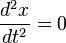$\frac{d^2x}{dt^2} = 0$

The component of acceleration along the vertical direction is$g$ downward, so we get: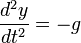$\frac{d^2y}{dt^2} = -g$

### Kinetics

We use the dynamics results and the initial conditions: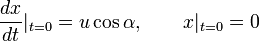$\frac{dx}{dt}|_{t = 0} = u \cos \alpha, \qquad x |_{t = 0} = 0$

to obtain: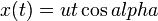$x(t) = ut \cos alpha$

Similarly, we get: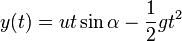$y(t) = ut \sin \alpha - \frac{1}{2}gt^2$

Also, the horizontal and vertical components of velocity are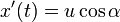$x'(t) = u\cos \alpha$ and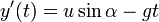$y'(t) = u \sin \alpha - gt$ respectively.

### Key quantities

We have the following formulas for key quantities:

Quantity Value Explanation
Time taken to attain maximum height$\frac{u\sin \alpha}{g}$ In the expression$y'(t) = u\sin \alpha - gt$, set$y'(t) = 0$.
Value of maximum height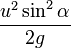$\frac{u^2\sin^2\alpha}{2g}$ Can be seen by plugging in time from above into the expression for$y(t)$. Can also be seen using a conservation of energy argument.
Time taken to hit the ground again$\frac{2u\sin \alpha}{g}$ In the expression$y(t) = ut \sin \alpha - \frac{1}{2}gt^2$, set$y(t) = 0$.
Horizontal distance traveled before hitting the ground again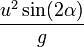$\frac{u^2\sin(2\alpha)}{g}$ Plug in time value above into expression for$x(t)$, use identity$\sin(2\alpha) = 2\sin \alpha \cos \alpha$

### Choosing the angle appropriately

We note from the above that:

• For a given initial speed$u$, the maximum vertical height, as well as maximum time taken to attain the maximum vertical height, occurs when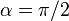$\alpha = \pi/2$, i.e., the projectile is fired vertically.
• For a given initial speed$u$, the maximum horizontal distance traversed occurs when$\alpha = \pi/4$.